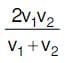# Important Questions Test: Motion & Time

## 20 Questions MCQ Test Online MCQ Tests for Class 7 | Important Questions Test: Motion & Time

Description
Attempt Important Questions Test: Motion & Time | 20 questions in 20 minutes | Mock test for Class 7 preparation | Free important questions MCQ to study Online MCQ Tests for Class 7 for Class 7 Exam | Download free PDF with solutions
QUESTION: 1

### A body covers half of its journey with a speed of 60 m/s and the other half with a speed of 40 m/s. Then average speed during whole journey is

Solution:

Average speed of body =where V= 60 m/s

V= 40 m/s

Average speed = 2 * 60 *40 / 100

= 4800/100

= 48 m/s

QUESTION: 2

### The rate of change of displacement with time is called

Solution:

Velocity is the rate of motion, speed or action. An example of velocity is a car driving at 75 miles per hour.

QUESTION: 3

### Which of the following is the basic unit of speed?

Solution:

Speed is the distance traveled per unit of time.It is denoted as m/s.

QUESTION: 4

A micro-second is equal to

Solution:

A microsecond is an SI unit of time equal to one millionth (0.000001 or 10−6 or ​1⁄1,000,000) of a second.

QUESTION: 5

If a pendulum is vibrating at 10 Cycles−1, its time period is

Solution:

Time period = time for one complete

oscillation=1/10 sec=0.1second

QUESTION: 6

Physical quantity which we can't obtain from a distance- time graph is

Solution:

A distance-time graph shows how far an object has travelled in a given time. Distance is plotted on the Y-axis (left) and Time is plotted on the X-axis.

QUESTION: 7

A body has an acceleration of4ms−2. What is its retardation?

Solution:

Retardation means negative acceleration.

QUESTION: 8

If the velocity of a body does not change with time, its acceleration is

Solution:

Velocity is the rate of change of position with respect to time, whereas acceleration is the rate of change of velocity.If velocity does not change then the acceleration would be zero.

QUESTION: 9

All of the following is a unit of speed except

Solution:

Pounds per square inch or p.s.i. is the unit of pressure. Pound is the unit weight and square inch is that of area. Therefore, p.s.i. cannot be the unit of speed.

QUESTION: 10

Which of the following does not show oscillatory motion?

Solution:

A fan shows circular motion.

QUESTION: 11

Which of the following statement(s) is/are correct?

Solution:

Symbols of all units are written in singular

QUESTION: 12

It is a part of wall clock and performs oscillatory motion. Its time period is fixed. It is

Solution:

Pendulums are used to regulate the movement of clocks because the interval of time for each complete oscillation, called the period, is constant.

QUESTION: 13

A body moving in a straight line is said to be in uniform motion, if

Solution:

It covers equal distances in equal intervals of time, how so ever small the interval may be

QUESTION: 14

Why is the average speed of a moving object same as the actual speed of the moving object in case of uniform motion in a straight line?

Solution:

Uniform motion is the motion when the object travels in a straight line and its velocity remains constant along that line as it covers equal distances in equal intervals of time.

QUESTION: 15

A graph is plotted showing the velocity of a car as a function of time. If the graph is a straight line, it means that

Solution:

If velocity isn't changing with time,then there is motion in the body with a constant velocity in a fixed direction but acceleration is Zero.

QUESTION: 16

The acceleration of a car that speeds up from 12 meters per second to 30 meters per second in 15 seconds is

Solution:

Acceleration = 30-12/15
=18/15
=1.2m/s

QUESTION: 17

A velocity-time graph gives (i) the distance (ii) the displacement (iii) the acceleration (iv) the speed

Solution:

A velocity-time graph shows changes in velocity of a moving object over time. The slope of a velocity-time graph represents acceleration of the moving object.

QUESTION: 18

Displacement is the

Solution:

Displacement is the shortest distance between initial and final positions.

QUESTION: 19

An object has travelled 10 km in 15 minutes, its displacement will be

Solution:

An object has traveled 10 km in 15 minutes; its displacement will be according to the direction it has followed.

QUESTION: 20

If an object covers equal distances in equal intervals of time, it is said to be in

Solution:

If an object covers equal distances in equal intervals of time, it is said to be in uniform motion.Use Code STAYHOME200 and get INR 200 additional OFF Use Coupon Code